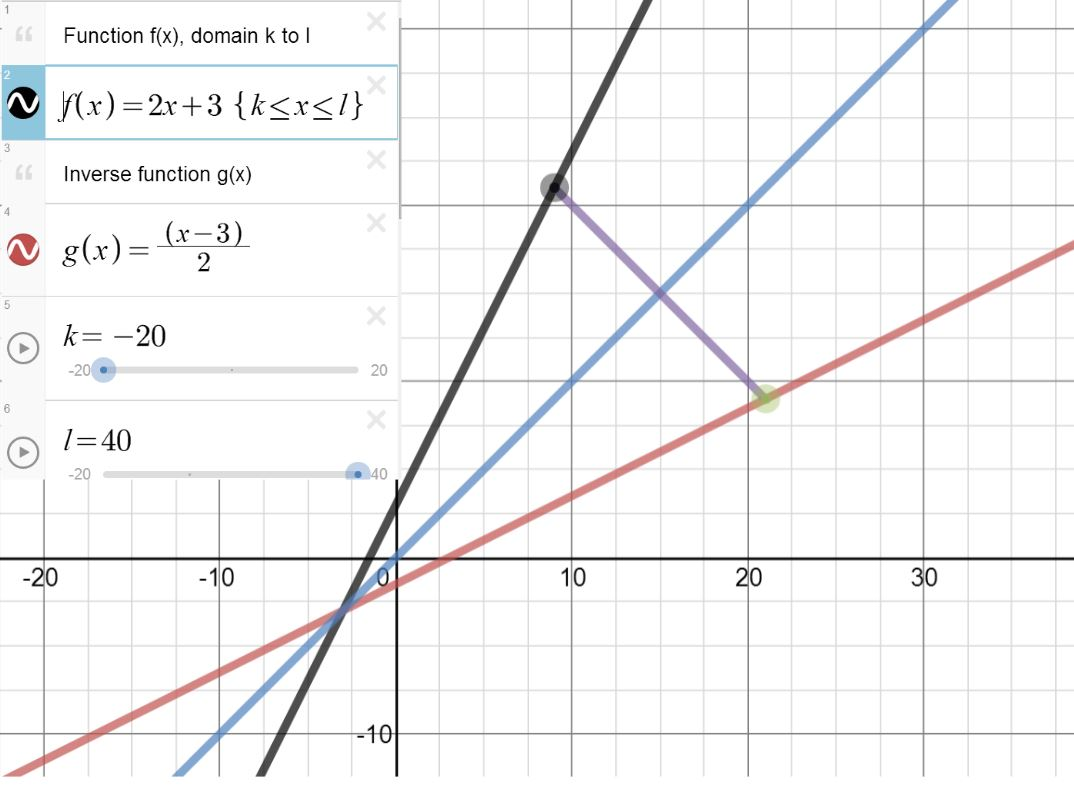# Functions

Thinking about teaching functions in the next few weeks (to UK Year 12 ages 16-17) I realised that I could use Desmos to illustrate composite functions; the following slideshow illustrates the syntax.

We can also use Desmos to illustrate a function and its inverse. To create the page below (select the image), I started with a graph already online illustrating the general case of a quadratic function and its inverse and simplified it. f(x) and g(x) can be changed to a different function and its inverse. Note that the domain of f(x) can be changed.Further examples: exponential function and basic quadratic (where we need to restrict the domain for an inverse function to exist).

Staying with Desmos, as I have mentioned before, the function notation is excellent for transformations:

It is also possible to define a function with more than one argument and use Desmos as a calculator

For some clear examples and a resource to point students to, Functions from The University of Plymouth Mathematics Support Materials is useful. The format used in this series makes the examples clear and all the exercises given have answers.

Other useful resources (requires Java) include the Wisweb applets, algebra arrows could be used to demonstrate functions and their inverses as shown in the following images.

This slideshow requires JavaScript.

1.goldenoj
•Colleen Young